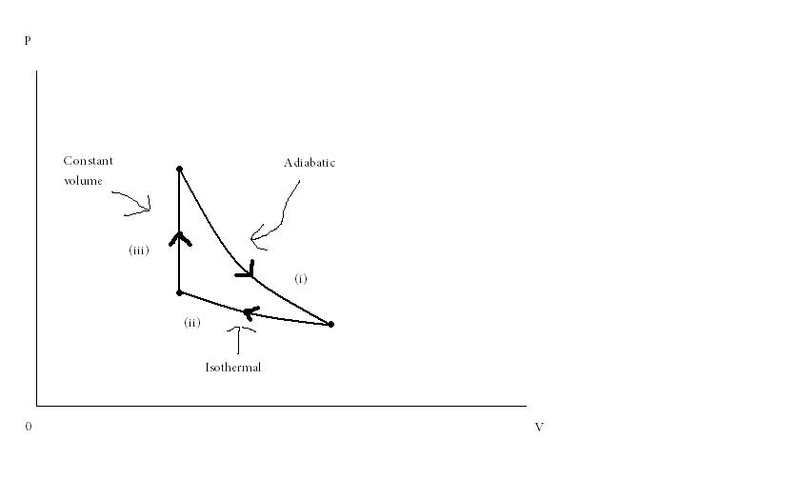# Efficiency of a heat engine in a reversible cycle

JSmith123

## Homework Statement

A heat engine operates in a reversible cycle with the following steps:(i) An ideal gas characterized by γ = 1.4 expands adiabatically, so that it cools from an initial temperature of 330°C to 30°C.
(ii) It is compressed isothermally until it reaches its initial volume.
(iii) It is then heated at constant volume until it reaches its initial temperature.

Calculate the efficiency of the cycle.
η =

Compare your answer above with the Carnot efficiency that corresponds to these temperatures.
η/η_Carnot =

## Homework Equations

η_Carnot = 1 - T_c/T_h

## The Attempt at a Solution

The answers are 0.30 and 60%. I know how to get the Carnot efficiency, but I'm not sure how to solve for the first answer. How do you find the efficiency with just 2 temperatures and a heat capacity ratio?

RTW69
Obviously the carnot eff= 50%

cycle efficiency=Work out/work in

Look first at the adiabatic process.The equation for work is just -n*R*(T_f-T_i)/(Y-1)

You can use the ratios of temperatures and volumes for adiabatic processes to find V_f/V_i that ratio is important because it is the same ratio needed for the isothermal process.

For the isothermal process W=-n*R*T*(V_f/V_i)

The cycle efficiency should be the ratio of isothermal work and adabatic work which is 70%, it should be 30% or 1-.7

There is a mistake somewhere, if some one finds it let me know!